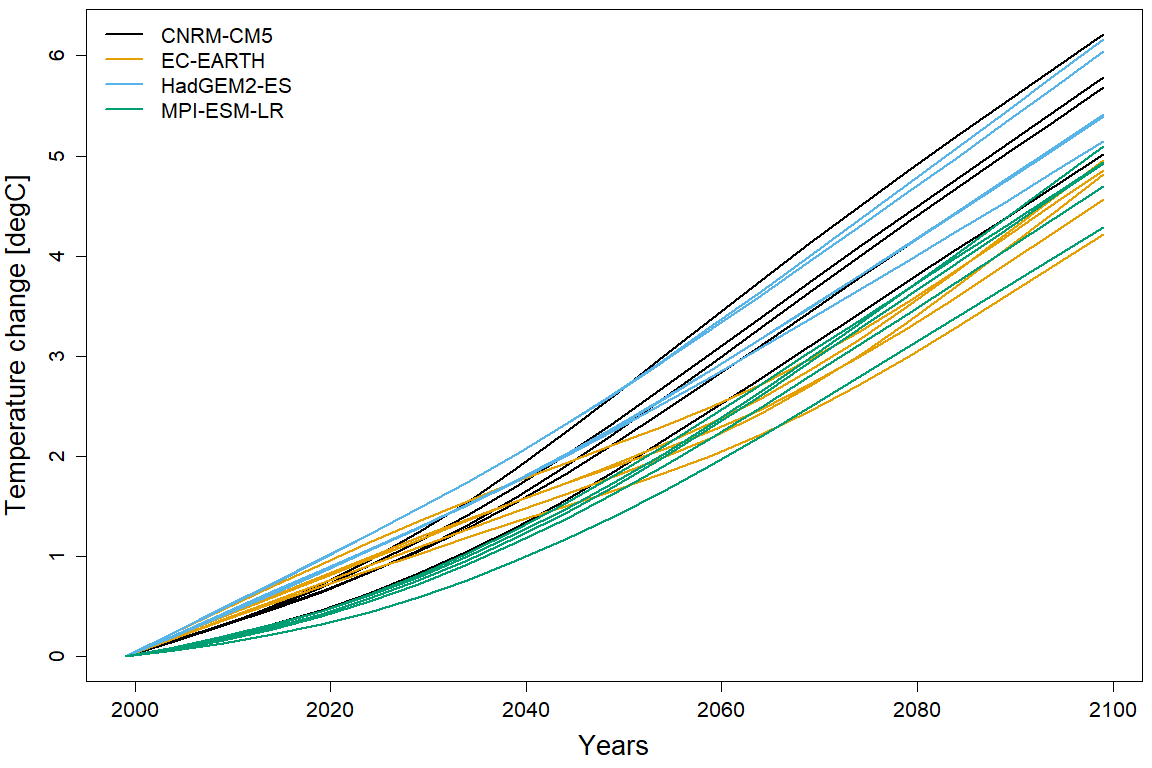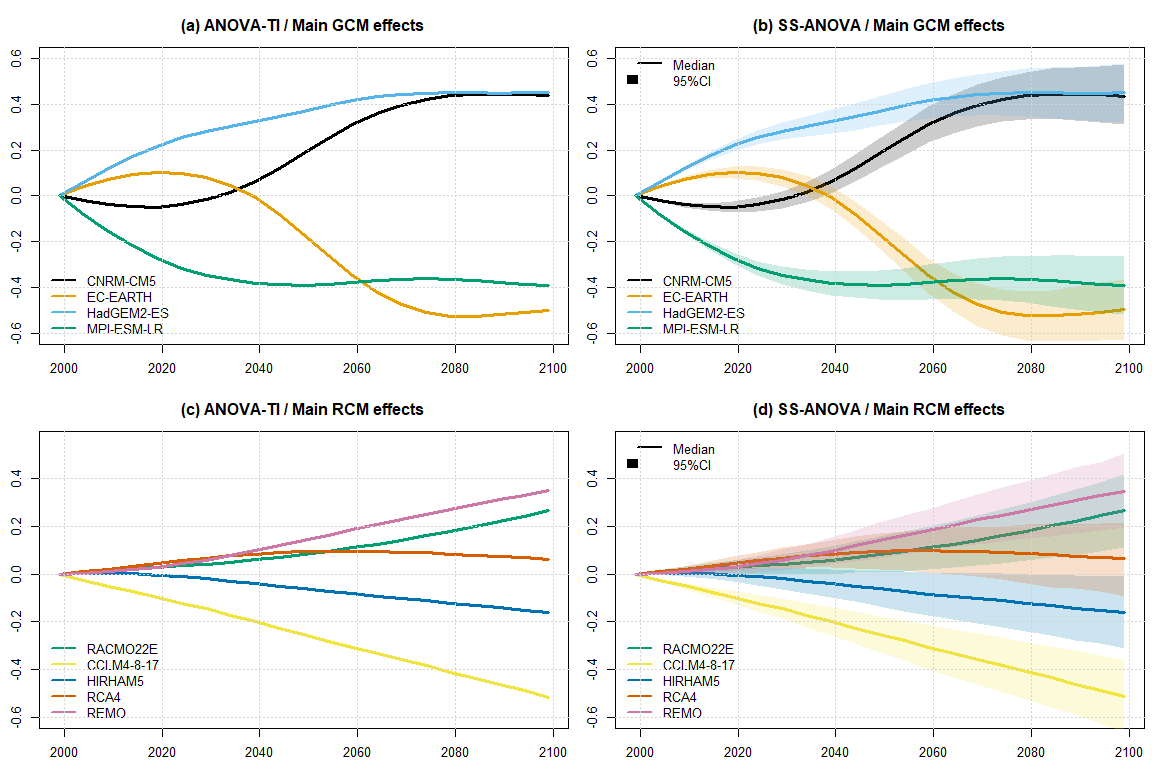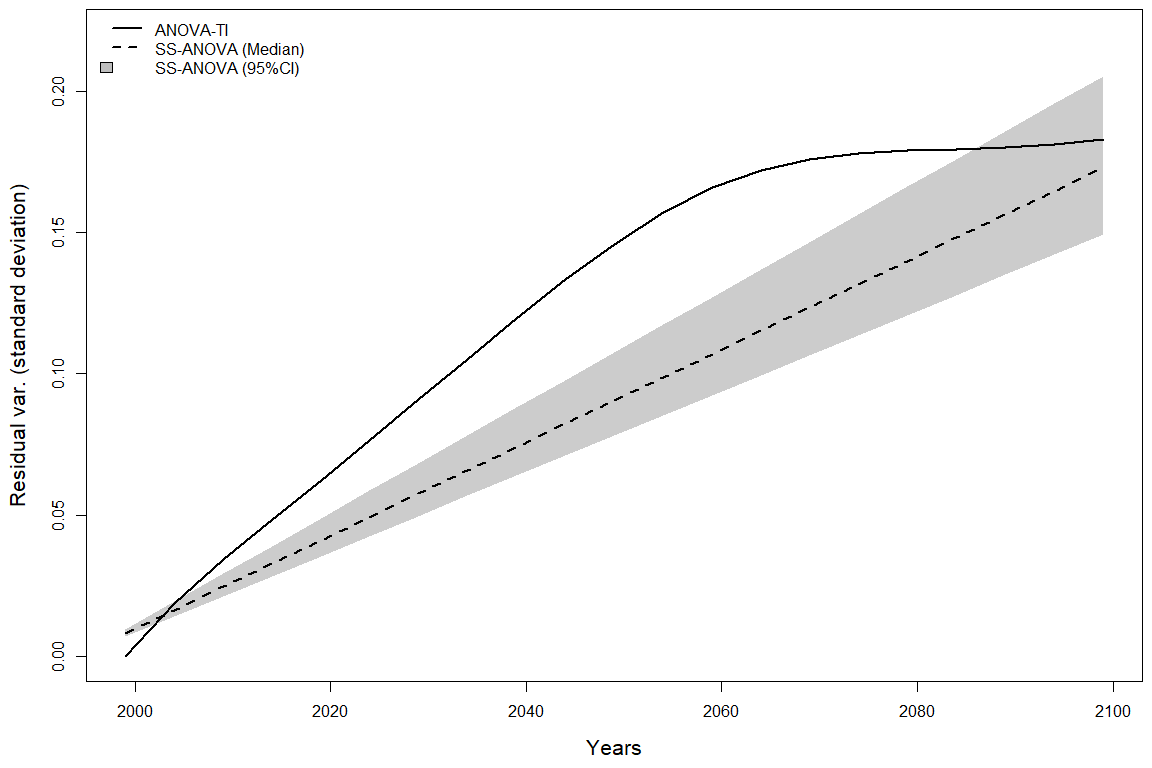# Application to an ensemble of regional climate projections

## Introduction

This vignette presents an application of a statistical framework which aims at partitioning uncertainty components in climate projections using smoothing splines. This approach is described in detail in a working report (Evin, 2022). These developments concern the assessment of uncertainties associated with future projections. Indeed, climate projections are important tools for our understanding of climate change impacts. Over the recent years, uncertainty in climate projections has been mostly explored and partitioned using Multiscenarios Multimodel Multimember Ensembles (MMEs) of transient climate projections. Various methods have been proposed for this, most of them based on an Analysis of Variance (ANOVA). The package qualypsoss implements a Smoothing-Spline ANOVA approach (SS-ANOVA) where the main effect of each climate model is represented as a smooth function of time. A Bayesian framework is proposed to handle heteroscedastic and autocorrelated residual errors between the climate change responses and the main additive effects modelled with cubic smoothing splines.

### Main reference

Evin, G. (2022) “Partitioning uncertainty components in climate projections using smoothing splines.” INRAE - UR ETNA, Grenoble, France, https://hal.archives-ouvertes.fr/hal-03720621.

## Ensemble of climate projections

The MME used in this study is composed of n=20 simulations obtained from the CMIP5-EUROCORDEX experiment for all combinations of 4 General Circulation Models (GCMs) and 5 Regional Climate Models (RCMs). Simulation chains are composed of historical runs for the periods 1971-2005, and of future runs for the period 2006-2099 obtained with the emission scenario RCP8.5. Our application focuses on mean temperature in winter averaged over the large Central Europe (CEU) region (land and sea points) considered in the IPCC SREX report which cover most of European countries above the 45th parallel north at the exception of Norway, Sweden, Finland, Denmark and United Kingdom.

The package QUALYPSO is loaded in order to provide a simple ANOVA method used as a benchmark in this work. This approach applies a linear ANOVA model for each time step and is denoted as ANOVA-TI.

In this study, the packages QUALYPSO and qualypsoss apply the same approach to obtain climate change responses. Climate responses $$\mathbf{\phi}_i,\, i=1,\dots,n=20$$ are first obtained using cubic smoothing splines implemented by the function smooth.spline to each simulation chain i. A high smoothing parameter (spar=1) is chosen in order to avoid including decennial variability into these fitted forced responses. For each simulation chain, we obtain climate change responses $$\mathbf{\phi}^*_i$$ as absolute differences between the climate responses obtained for future and reference years, the year $$c=1999$$ being retained as the reference year.

Figure 1 illustrates the climate change responses $$\mathbf{\phi}^*_i$$. The absolute temperature changes are equal to 0 in 1999 by construction, and gradually increase up to between 4°C and 6°C in 2099. The different simulations for each GCM clearly show that some GCMs lead to higher temperature changes (CNRM-CM5 and HadGEM2-ES) than the other (EC-EARTH, MPI-ESM-LR).

library(QUALYPSO)

# Projections of mean winter temperature for 20 simulations of 129 years are contained in the matrix Y
#[20 x 129]
dim(Y)
#>   20 129

# Corresponding years are provided in the vector X_time_vec
X_time_vec
#>    1971 1972 1973 1974 1975 1976 1977 1978 1979 1980 1981 1982 1983 1984 1985
#>   1986 1987 1988 1989 1990 1991 1992 1993 1994 1995 1996 1997 1998 1999 2000
#>   2001 2002 2003 2004 2005 2006 2007 2008 2009 2010 2011 2012 2013 2014 2015
#>   2016 2017 2018 2019 2020 2021 2022 2023 2024 2025 2026 2027 2028 2029 2030
#>   2031 2032 2033 2034 2035 2036 2037 2038 2039 2040 2041 2042 2043 2044 2045
#>   2046 2047 2048 2049 2050 2051 2052 2053 2054 2055 2056 2057 2058 2059 2060
#>   2061 2062 2063 2064 2065 2066 2067 2068 2069 2070 2071 2072 2073 2074 2075
#>  2076 2077 2078 2079 2080 2081 2082 2083 2084 2085 2086 2087 2088 2089 2090
#>  2091 2092 2093 2094 2095 2096 2097 2098 2099

# GCM and RCM models GCM et RCM corresponding to the 20 simulations are given in scenAvail (data.frame
# with two columns "GCM" et "RCM" and 20 lines corresponding to the 20 simulations). These 20 simulations
# correspond to 4 GCMs downscaled with 5 RCMs
dim(scenAvail)
#>  20  2
scenAvail
#>           GCM        RCM
#> 1    CNRM-CM5   RACMO22E
#> 2    CNRM-CM5 CCLM4-8-17
#> 3    CNRM-CM5    HIRHAM5
#> 4    CNRM-CM5       RCA4
#> 5    CNRM-CM5       REMO
#> 6    EC-EARTH   RACMO22E
#> 7    EC-EARTH CCLM4-8-17
#> 8    EC-EARTH    HIRHAM5
#> 9    EC-EARTH       RCA4
#> 10   EC-EARTH       REMO
#> 16 MPI-ESM-LR   RACMO22E
#> 17 MPI-ESM-LR CCLM4-8-17
#> 18 MPI-ESM-LR    HIRHAM5
#> 19 MPI-ESM-LR       RCA4
#> 20 MPI-ESM-LR       REMO
apply(scenAvail,2,unique)
#> $GCM #>  "CNRM-CM5" "EC-EARTH" "HadGEM2-ES" "MPI-ESM-LR" #> #>$RCM
#>  "RACMO22E"   "CCLM4-8-17" "HIRHAM5"    "RCA4"       "REMO"

# We define a vector of future years to reduce the dimension of the SS-ANOVA treatment
Xfut_time = seq(from=1999,to=2099,by=5)

# This list contains the different objects processed by the ANOVA methods
lInput = list(Y=Y,vecYears=X_time_vec,Xref=1999,Xfut=Xfut_time,scenAvail=scenAvail)

# in listOption, we can specify the type of change (absolute "abs" or relative "rel")
listOption = list(typeChangeVariable="abs")

# The call to QUALYPSO extracts the climate change responses and applies a simple
# ANOVA for each future year
QUALYPSOOUT = QUALYPSO(Y=lInput$Y,X=lInput$vecYears,scenAvail=lInput$scenAvail, Xref=lInput$Xref,Xfut=lInput$Xfut, listOption=listOption) # phiStar is matrix 20 x 21 (n=20 simulations x nFut=21 future time steps) containing the climate change responses phiStar = QUALYPSOOUT$CLIMATEESPONSE$phiStar # vec.GCM contains the vector of the 4 different GCMs vec.GCM = unique(scenAvail$GCM)

# Figure 1
par(mar=c(4.5,4.5,0.5,0.5))
plot(-1,-1,xlim=range(lInput$Xfut),ylim=range(phiStar),cex.axis=1.3, xlab="Years",ylab="Temperature change [degC]",cex.lab=1.7) for(i in 1:nrow(scenAvail)){ i.GCM = which(scenAvail$GCM[i]==vec.GCM)
lines(lInput$Xfut,phiStar[i,],lwd=2,col=mycol.GCM[i.GCM]) } legend('topleft',bty='n',cex=1.3,lty=1,lwd=2,col=mycol.GCM,legend=vec.GCM)## SS-ANOVA The Bayesian SS-ANOVA approach is applied by sampling 6,000 MCMC draws of all unknown quantities, i.e. the smoothing spline effects, the residual variability, the autocorrelation of the residual errors and the smoothing parameters. Because the climate change responses evolve smoothly, the climate change responses are highly autocorrelated. The SS-ANOVA framework formalizes the representation of the autocorrelation of the residual errors with an AR1 model, together with their heteroscedasticity with a linear evolution of their standard deviation. # we load the package qualypsoss library(qualypsoss,exclude=c("Y","scenAvail")) # the different options listed here specify: absolute change; 1,000 MCMC draws for the burn-in period, #5,000 MCMC draws to represent the posterior distributions, MCMC draws are returned in the output #(returnMCMC=TRUE), a unique climate change response is used (uniqueFit=TRUE) with the smoothing parameter #spar=1, we consider an AR1 process for the autocorrelation of the residual errors, and a linear evolution #of their standard deviations. listOption = list(typeChangeVariable="abs",nBurn=1000,nKeep=5000,returnMCMC=TRUE, uniqueFit=TRUE,spar=1,type.temporal.dep="AR1",type.hetero="linear") # Call to the main function of the package qualypsoss, see ?QUALYPSOSS for further information on the #inputs and possible options QUALYPSOSSOUT = QUALYPSOSS(ClimateProjections=t(lInput$Y),
scenAvail=lInput$scenAvail, vecYears = lInput$vecYears,
predContUnique = lInput$Xfut, iCpredCont = which(lInput$vecYears==lInput$Xref), # 1999 iCpredContUnique = which(lInput$Xfut==lInput$Xref), # 1999 listOption=listOption) ## Main climate effects Figure 2 compares the GCM and RCM main effects for the ANOVA-TI and SS-ANOVA approaches. Concerning the SS-ANOVA approach, median estimated effects are indicated by thick lines. The uncertainty related to the estimation of each individual effect is indicated by 95% credible intervals obtained from the corresponding posterior distributions. Mean estimated effects obtained with the two approaches are very similar. These results highlight the discrepancies between two groups of GCMs: CNRM-CM5 and HadGEM2-ES versus EC-EARTH and MPI-ESM-LR, the former leading to higher temperature changes than the latter. # the functions plotQUALYPSOeffect and plotQUALYPSOSSeffect directly display the evolution of the main #effects from the two ANOVA approaches par(mar=c(2.5,2.5,3,0.5),mfrow=c(2,2)) plotQUALYPSOeffect(QUALYPSOOUT,nameEff = "GCM",col = mycol.GCM,lim = c(-0.6,0.6), xlab="",ylab="",main="(a) ANOVA-TI / Main GCM effects",cex.lab=1.3) grid() plotQUALYPSOSSeffect(QUALYPSOSSOUT,iEff=1,col = mycol.GCM,lim = c(-0.6,0.6), xlab="",ylab="",main="(b) SS-ANOVA / Main GCM effects",cex.lab=1.3) grid() plotQUALYPSOeffect(QUALYPSOOUT,nameEff = "RCM",col = mycol.RCM,lim = c(-0.6,0.55), xlab="",ylab="",main="(c) ANOVA-TI / Main RCM effects",cex.lab=1.3) grid() plotQUALYPSOSSeffect(QUALYPSOSSOUT,iEff=2,col = mycol.RCM,lim = c(-0.6,0.55), xlab="",ylab="",main="(d) SS-ANOVA / Main RCM effects",cex.lab=1.3) grid()## Residual variance Figure 4 shows the standard deviation of the residual errors obtained with the benchmark approach ANOVA-TI and the SS-ANOVA approach proposed in this study. The ANOVA-TI approach applies a linear model for each year and does not assume a particular form of evolution for the standard deviation of the residual errors, whereas this evolution is linear for the SS-ANOVA approach. The residual variability obtained with ANOVA-TI seems to increase linearly until 2060 and becomes constant afterwards, which creates a discrepancy with the SS-ANOVA approach. # we extract here the standard deviations of the residual errors (square root of the variances) sigRes.QUA = sqrt(QUALYPSOOUT$RESIDUALVAR$MEAN) sigRes.SSinf = sqrt(QUALYPSOSSOUT$BAYES$RESIDUALVAR[2,]) sigRes.SSmed = sqrt(QUALYPSOSSOUT$BAYES$RESIDUALVAR[4,]) sigRes.SSsup = sqrt(QUALYPSOSSOUT$BAYES$RESIDUALVAR[6,]) par(mar=c(4.5,4.5,0.5,0.5),mfrow=c(1,1)) plot(lInput$Xfut,sigRes.QUA,ylim=c(0,0.22),type="l",lwd=2,cex.lab=1.3,
xlab="Years",ylab="Residual var. (standard deviation)")
polygon(x = c(lInput$Xfut,rev(lInput$Xfut)),y=c(sigRes.SSinf,rev(sigRes.SSsup)),
col=adjustcolor("black", alpha.f = 0.2), lty = 0)
lines(lInput\$Xfut,sigRes.SSmed,ylim=c(0,0.15),lwd=2,lty=2)
legend("topleft", bty = "n", fill = c(NA, NA, "grey"), lwd = c(2, 2, NA), lty = c(1, 2, NA),
border = c(NA,NA,"black"), col = c("black","black", NA),
legend = c("ANOVA-TI","SS-ANOVA (Median)","SS-ANOVA (95%CI)"))